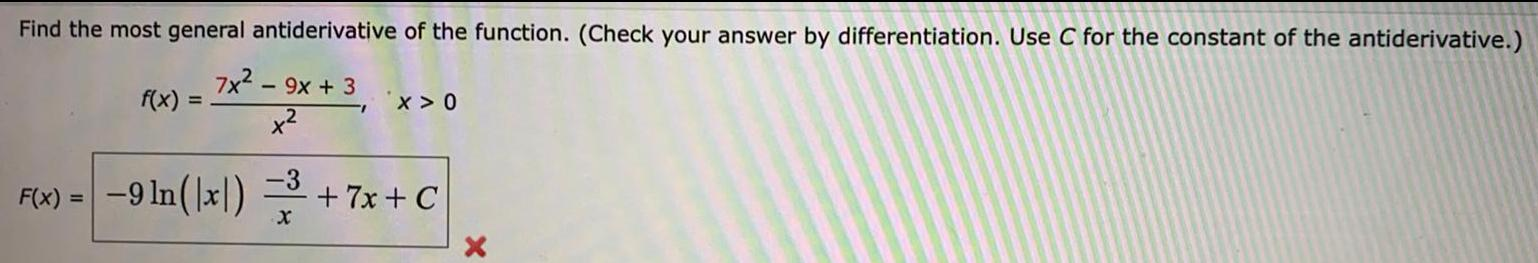Question:

# Find the most general antiderivative of the function. (CheckFind the most general antiderivative of the function. (Check your answer by differentiation. Use C for the constant of the antiderivative.) 7x² - 9x + 3 x² F(x) = f(x): = X>0 -3 -9 ln(x)=³ +7x+C